# Matrices and Determinant

Welcome to class!

In today’s class, we will be talking about matrices and determinant. Enjoy the class!

### Matrices and Determinant#### Matrix

A matrix is defined as an ordered rectangular array of numbers. They can be used to represent systems of linear equations, as will be explained below.

Here are a couple of examples of different types of matrices:If A and B above are matrices of the same type, then the sum is found by adding the corresponding elements aij + bij.

Here is an example of adding A and B together.• ##### Subtraction:

If A and B are matrices of the same type, then the subtraction is found by subtracting the corresponding elements aij − bij.

Here is an example of subtracting matrices.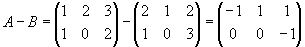• ##### Matrix multiplication:

When the number of columns of the first matrix is the same as the number of rows in the second matrix then matrix multiplication can be performed.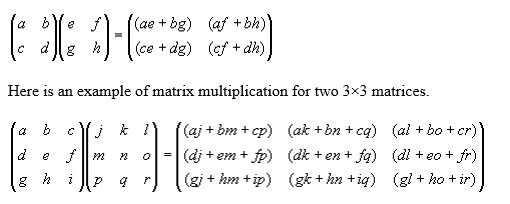Now let’s look at the n × n matrix case, Where A has dimensions m × n, B has dimensions n×p. Then the product of A and B is the matrix C, which has dimensions m×p. The ijth element of matrix C is found by multiplying the entries of the ith row of A with the corresponding entries in the jth column of B and summing the n terms. The elements of C are: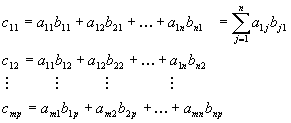Note: That A×B is not the same as B×A

• ##### Transpose of matrices:

The transpose of a matrix is found by exchanging rows for columns i.e. Matrix A = (aij) and the transpose of A is:

AT = (aji) where j is the column number and i is the row number of matrix A.

For example, the transpose of a matrix would be: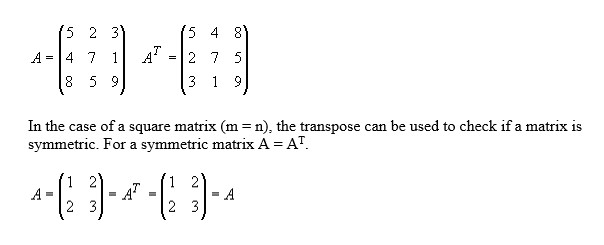• ##### The determinant of a matrix:

Determinants play an important role in finding the inverse of a matrix and in solving systems of linear equations. In the following, we assume we have a square matrix (m = n). The determinant of a matrix A will be denoted by det(A) or |A|.

Firstly, the determinant of a 2×2 and 3×3 matrix will be introduced, then the n×n case will be shown.

###### The determinant of a 2×2 matrix:

Assuming A is an arbitrary 2×2 matrix A, where the elements are given by:###### The determinant of a 3×3 matrix

The determinant of a 3×3 matrix is a little trickier and is found as follows (for this case assume A is an arbitrary 3×3 matrix A, where the elements are given below).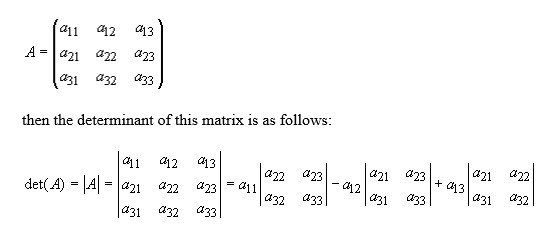• ##### The inverse of a matrix:

Assuming we have a square matrix A, which is non-singular (i.e. det(A) does not equal zero), then there exists an n×n matrix A-1 which is called the inverse of A, such that this property holds:

AA-1 = A-1A = I, where I is the identity matrix.

###### The inverse of a 2×2 matrix:

Take for example a 2×2 Matrix A whose determinant (ad − bc) is not equal to zero.

######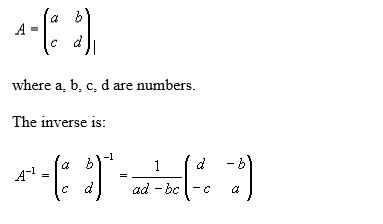Inverse matrix method:

The inverse matrix method uses the inverse of a matrix to help solve a system of equations, such as the above Ax = b. By pre-multiplying both sides of this equation by A-1 gives:

x = A-1

So, by calculating the inverse of the matrix and multiplying this by the vector b we can find the solution to the system of equations directly. And from earlier we found that the inverse is given by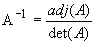From the above, the existence of a solution depends on the value of the determinant of A. There are three cases:

1. If the det(A) does not equal zero, then solutions exist using x = A-1 b
2. The det(A) is zero and b=0 then the solution will be not be unique or does not exist.
3. If the det(A) is zero and b=0 then the solution can be x = 0 but as with 2. is not unique or does not exist.

Looking at two equations we might have that

ax + by = c

dx + ey = f

Written in matrix form would look likeand by rearranging we would get that the solution would look likeIn our next class, we will be talking about Linear and Quadratic Equations. We hope you enjoyed the class.

Should you have any further question, feel free to ask in the comment section below and trust us to respond as soon as possible.

How Can We Make ClassNotesNG Better - CLICK to Tell Us💃